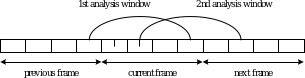5.1.5 Spectral analysis

26.4453GPPCodec for Enhanced Voice Services (EVS)Detailed algorithmic descriptionRelease 15TS

Spectral analysis is used in the encoder for signal activity detection (SAD) and signal classification functions. The discrete Fourier transform (DFT) is used to perform the spectral analysis and spectral energy estimation.

5.1.5.1 Windowing and DFT

The frequency analysis is done twice per frame using 256-point fast Fourier transform (FFT) with a 50% overlap. The centre of the first window is placed 96 samples past the beginning of the current frame. The centre of the second window is placed 128 samples farther, i.e., in the middle of the second subframe of the current frame. A square root of a Hanning window (which is equivalent to a sine window) is used to weight the input signal for the frequency analysis. The square root Hanning window is given by

(18)

where = 256 is the size of FFT analysis. Note that only half of the window is computed and stored since it is symmetric (from 0 to ).Figure 5: Relative positions of the spectral analysis windows

The windowed signal for both spectral analyses is obtained as:

(19)

whereis the pre-emphasized input signal (is the first sample in the current frame). The superscripts  and  used to denote the first and the second frequency analysis, respectively, are dropped for simplicity. An FFT is performed on both windowed signals to obtain two sets of spectral parameters per frame:

(20)

The output of the FFT provides the real and the imaginary parts of the spectrum denoted as, and , . Note, that corresponds to the spectrum at 0 Hz (DC) and corresponds to the spectrum at 6400 Hz. The spectrum at these points is only real-valued and usually ignored in the subsequent analysis.

After the FFT analysis, the resulting spectrum is divided into critical bands  using the intervals having the following limits (20 bands in the frequency range 0-6400 Hz):

Table 2: Critical bands

 band 0 0 100 2 1 100 200 2 2 200 300 2 3 300 400 2 4 400 510 2 5 510 630 2 7 630 770 3 6 770 920 3 8 920 1080 3 9 1080 1270 4 10 1270 1480 4 11 1480 1720 5 12 1720 2000 6 13 2000 2320 6 14 2320 2700 8 15 2700 3150 9 16 3150 3700 11 17 3700 4400 14 18 4400 5300 18 19 5300 6350 21

The 256-point FFT results in a frequency resolution of 50 Hz (i.e., 6400/128 Hz). Thus, after ignoring the DC component of the spectrum, the number of frequency bins per critical band are given in the last column, denoted .

5.1.5.2 Energy calculations

The spectral analysis module also calculates several energy-related parameters. For example, an average energy per critical band is computed as

(21)

whereandare, respectively, the real and the imaginary parts of the-th frequency bin and is the index of the first bin in the ith critical band given by={1, 3, 5, 7, 9, 11, 13, 16, 19, 22, 26, 30, 35, 41, 47, 55, 64, 75, 89, 107}. Furthermore, energy per frequency bin,, is calculated as

(22)

Finally, the spectral analysis module computes the average total energy for both FFT analyses in a 20 ms frame by summing the average critical band energies. That is, the spectrum energy for the first spectral analysis window is computed as

(23)

and, similarly, the second frame energy, denoted as.

The total frame energy (in dB) is computed as the average of the two frame energies. That is

(24)

The total energy per frequency bin (power spectrum) is calculated as

(25)

The output parameters of the spectral analysis module (both spectral analyses), that is the average energy per critical band, the energy per frequency bin and the total energy in dB, are used in several subsequent functions.

Note that, for narrow band inputs sampled at 8 kHz, after sampling conversion to 12.8 kHz, there is no content at both ends of the spectrum. Thus, the lowest critical band as well as the last three critical bands are not considered in the computation of output parameters (only bands from are considered).

In addition to the absolute frame energy, calculated in (24), relative energy of the frame is calculated as the difference between the total frame energy in dB and the long-term active signal energy. The relative frame energy is given by

(26)

The long-term active signal energy is updated only during active frames (explained in subclause 5.1.12.5). Note that the long-term active signal energyis updated only after the signal activity detection module.